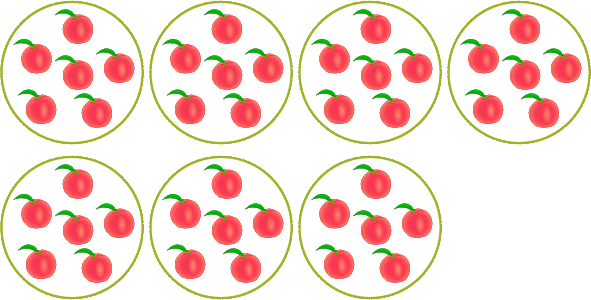# 7 times table quiz - Multiply by 7 test

## 7 times table test

There are a mixture of diverse and exigent x7 multiplication games quizzes in our 7 times table quiz, ranging from (7 x 1) to (7 x 12).
Multiply by 7 test as a game element will reinforce a quick and easy mastery of multiplication by 7.

## Multiplication Methods Activities

### What you need to know about 7 times table

In order to score a 100/100 in the 7 times table test, you need to use very simple and effective methods to easily obtain correct answers when multiplying by 7.

As an appropriate method, we can use the equal groups method to obtain our product.

### Example - obtain correct answer for 7 times table test

Here, you are required to choose the correct answer from the given ones, in response to the given multiplication sentence of our 7 times table test.

Multiply

7 x 5 = ?

Answer: [ 25, 30, 35 ]

We will quickly obtain our answer if we use the equal group method.

• Firstly, form 7 groups of 5 equal items.

Form 7 groups of 5 apples